• k近邻算法python
千次阅读
2022-05-04 21:01:52

## 机器学习算法 | Python实现k-近邻算法

### 基本介绍

KNN，全名k近邻算法。是⼀种⾮常简单有效的机器学习算法。KNN是通过计算不同特征值距离作为分类依据，即计算⼀个待分类对象不同​特征值与样本库中每⼀个样本上对应特征值的差值，将每个维度差值求和也就得到了该组数据与样本之间的距离，⼀般使⽤欧式距离进⾏计算，通过对所有样本求距离，最终得到离待分类对象最近的K个样本，将这K个点作为分类依据。

• 优点：精度高、对异常值不敏感、无数据输入假定,对数据的特征类型没有明确的要求；
• 缺点：时间复杂度高、空间复杂度高；

适用数据范围：数值型和标称型；
关键点：k值的选取，k值为10以内的奇数（奇数是为了避免出现打成平⼿的情况），也可以通过交叉验证的⽅式来选取得到合适的k值；

### 工作原理

• 存在一个样本数据集合，也称作训练样本集python 近邻算法 sklearn 机器学习
更多相关内容
• K近邻算法（或简称kNN）是易于理解和实现的算法，而且是你解决问题的强大工具。 什么是kNN kNN算法的模型就是整个训练数据集。当需要对一个未知数据实例进行预测时，kNN算法会在训练数据集中搜寻k个最相似实例。对...
• K近邻算法是对事物进行分类的机器学习算法。简单来说就是给定一组样本，对新的输入样本，在已有样本中找到与该实例最邻近的K个例子， 当这K个例子的大多属于某个类别时，就把该输入样本分类到这个类之中。 举例来...
• 一、概述

K近邻算法是对事物进行分类的机器学习算法。简单来说就是给定一组样本，对新的输入样本，在已有样本中找到与该实例最邻近的K个例子， 当这K个例子的大多属于某个类别时，就把该输入样本分类到这个类之中。

举例来说，小说有许多不同种类，暂且分成言情小说和玄幻小说，通过统计小说中的恋爱情节和打斗情节得出一组数据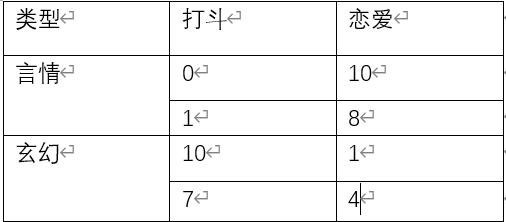当我们把该数据通过图表显示

此时，当有一部小说X的打斗情节为6，恋爱情节为2，会属于哪一类小说？（五角星代表小说X）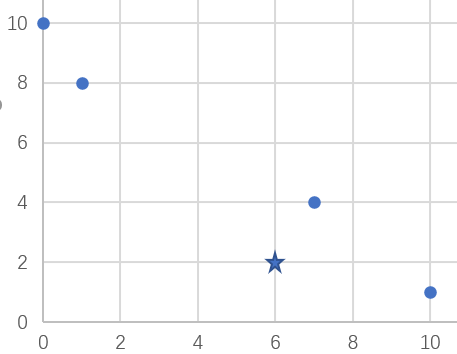计算小说X的数据到样本数据的距离，设k为3，则需要找到距离最小的三个点，当某一类更多时将小说X归于该类小说。这就是通过计算距离总结k个最邻近类并按照少数服从多数原则分类的K近邻算法。

• 二、算法原理介绍

以上面数据为例，打斗情节为x，恋爱情节为y，通过欧氏距离公式  计算该点与各个样本数据的距离，当然实际需要的数据有很多是多维的，但如三维的话可以在根号中加入z轴差的平方，也可以计算距离

现在再计算各个数据的距离，可以得到四个点的距离，将k定为3，则取其中最小的三个数，显而易见这三个数中有两个属于玄幻类，那么小说X属于玄幻小说。

• 三、算法实现

    trainData - 样本数据
testData - X数据
labels - 分类

def knn(trainData, testData, labels, k):

rowSize = trainData.shape # 计算样本数据的行数

diff = tile(testData, (rowSize, 1)) - trainData
# 计算样本数据和X数据的差值

sqrDiff = diff ** 2
sqrDiffSum = sqrDiff.sum(axis=1) # 计算差值的平方和

distances = sqrDiffSum ** 0.5 # 计算距离

sortDistance = distances.argsort()

count = {}

for i in range(k):
vote = labels[sortDistance[i]]
count[vote] = count.get(vote, 0) + 1
# 对所得距离从低到高进行排序
sortCount = sorted(count.items(), key=operator.itemgetter(1), reverse=True)
# 对类别出现的频数从高到低进行排序

return sortCount # 返回出现频数最高的类别
• 四、测试结果

trainData = np.array([[0, 10], [1, 8], [10, 1], [7, 4]])
labels = ['言情', '言情', '玄幻', '玄幻']
testData = [6, 2]
X = knn(trainData, testData, labels, 3)
print(X)

执行上列代码后得到结果为玄幻，与计算的相同。

当然实际上该算法不可能100%准确，还是会有不少的偏差，要更接近准确还需要更多的实验样本来减少可能的偏差。

展开全文python 机器学习
• 本篇文章，想向各位介绍一个非常易于实现的机器学习算法：k-近邻算法，众所周知，k-近邻算法是非常常用的分类算法，现在给出k-近邻算法的官方解释：在一个样本数据集，也称作训练样本集，并且样本中每个数据都存在...

本篇文章，想向各位介绍一个非常易于实现的机器学习算法：k-近邻算法，众所周知，k-近邻算法是非常常用的分类算法，现在给出k-近邻算法的官方解释：在一个样本数据集，也称作训练样本集，并且样本中每个数据都存在标签，即我们知道样本集中每一数据与所属分类的对应关系，输入没有标签的新数据后，将新数据的每个特征与样本集中的数据对应的特征进行比较，然后算法提取样本集中特征最相似的数据(最近邻)的分类标签。一般来说，我们只选择样本集中前k个最相似的数据，这就是k-近邻算法中k的出处，通常k是不大于20的整数，最后，选择k个最相似的数据中出现次数最多的分类，作为新数据的分类。通过寻找近邻来辅助分类可以提升分类的效率，简化分类的流程。

现在来介绍k-近邻算法的一般流程：

(1)收集数据：比如问卷法，观察法等等。

(2)准备数据：距离计算所需的数值，最好是结构化的数据格式。

(3)分析数据：聚类分析、因子分析、相关分析、对应分析、回归分析、方差分析等。

(4)训练算法：在k-近邻中一般不用。

(5)测试算法：计算错误率。

(6)使用算法：首先需要输入样本数据和结构化的输出结果，然后运行k-近邻算法判定输入数据分别属于哪个分类，最后应用对计算出的分类执行后续的处理。

介绍完了流程，我们开始导入数据，这次导入数据的代码环境依然选择python，首先我们需要有kNN.py的文件,关于kNN的源码，推荐各位上CodeForge网站上下载，该网站有很多有用的源码。

有了kNN的源码之后，我们可能还要编写一些基本的通用函数，在源码中增加下面的代码：

from numpy import *

Import operator

def createDataSet():

group = array([[1.0,1.1],[1.0,1.0],[0,0],[0,0.1]])

labels = [‘A’,’A’,’B’,’B’]

return group ,labels

在上面的代码中，我们导入了两个模块：第一个是科学计算包Numpy;第二个是运算符模块，k-近邻算法执行排序操作时将使用这个模块提供的函数。

现在我们来实施kNN算法，为每组数据分类，先给出其伪代码如下：

对未知类别属性的数据集中的每个点依次执行以下操作：

(1)计算已知类别数据集中的每个点与当前点之间的距离：

(2)按照距离递增次序排序；

(3)选取与当前距离最小的k个点；

(4)确定前k个点所在类别的出现频率；

(5)返回前k个点出现频率最高的类别作为当前点的预测分类。

K_近邻算法的python实现如下：

def classify0(inX,dataSet,labels,k):

dataSetSize = dataSet.shape

diffMat = tile(inX,(dataSetSize,1))-dataSet

sqDiffMat = diffMat**2

sqDistances = sqDiffMat.sum(axis=1)

distance = sqDistance**0.5

sortedDistIndicies = distance.argsort()

classCount = {}

for i in range(k):

voteIlabel = labels[sortedDistIndicies[i]]

classCount[voteIlabel] = classCount.get(voteIlabel,0) + 1

sortedClassCount = sorted(classCount.iteritems),key = operator.itemgetter(1),reverse = True)

return sortedClassCount

以上代码的目的主要是为了计算数据中的点与当前点的距离，根据计算的的距离结果，选择距离最小的k个点，随后对这k个点按照从大到小进行排序。(距离计算主要是欧式距离)

为了预测数据所在分类，在python提示符中输入下列命令：

kNN.classify0([0,0],group,labels,3)

以上是我们用python构造的第一个分类器，这个分类器可以完成很多任务，我们初步完成了构造分类算法。之后的文章中，将会给给位介绍一下k近邻算法的案例，敬请期待～

作者：徐永瑞 Python爱好者社区专栏作者，请勿转载，谢谢。

出处：k-近邻算法的Python实现

配套视频教程：Python3爬虫三大案例实战分享：猫眼电影、今日头条街拍美图、淘宝美食 Python3爬虫三大案例实战分享

公众号：Python爱好者社区(微信ID：python_shequ)，关注，查看更多连载内容。

加小编个人微信：tsdatajob ，跟作者互动，一起探讨。

展开全文• 用官方的话来说，所谓K近邻算法，即是给定一个训练数据集，对新的输入实例，在训练数据集中找到与该实例最邻近的K个实例（也就是上面所说的K个邻居）， 这K个实例的多数属于某个类，就把该输入实例分类到这个类中。...
• 主要介绍了Python机器学习k-近邻算法(K Nearest Neighbor),结合实例形式分析了k-近邻算法的原理、操作步骤、相关实现与使用技巧,需要的朋友可以参考下
• 2. 算法原理 2.1 算法优缺点 2.2 算法参数 2.3 变种 3.算法中的距离公式 4.案例实现 4.1 导入相关库 4.2 读取数据 4.3 读取变量名 4.4 定义X,Y数据 4.5 分离训练集和测试集 4.6 计算欧式距离 4.7可视化距离矩阵 4.8 ...

目录

1. 基本定义

2. 算法原理

2.1 算法优缺点

2.2 算法参数

2.3 变种

3.算法中的距离公式

4.案例实现

4.1 导入相关库

4.2 读取数据

4.3 读取变量名

4.4 定义X,Y数据

4.5 分离训练集和测试集

4.6 计算欧式距离

4.7 可视化距离矩阵

4.8 预测样本

4.9 查看正确率

4.10 交叉验证

5. scikit-learn的算法实现

5.1 对上述的再次实现：

5.2 另一种实现方式

## 1. 基本定义

k最近邻(k-Nearest Ne ighbor)算法是比较简单的机器学习算法。它采用测量不同特征值之间的距离方法进行分类。它的思想很简单：如果一个样本在特征空间中的多个最近邻(最相似〉的样本中的大多数都属于某一个类别，则该样本也属于这个类别。第一个字母k可以小写，表示外部定义的近邻数量。

简而言之，就是让机器自己按照每一个点的距离，距离近的为一类。

## 2. 算法原理

knn算法的核心思想是未标记样本的类别，由距离其最近的k个邻居投票来决定。
具体的，假设我们有一个已标记好的数据集。此时有一个未标记的数据样本，我们的任务是预测出这个数据样本所属的类别。knn的原理是，计算待标记样本和数据集中每个样本的距离，取距离最近的k个样本。待标记的样本所属类别就由这k个距离最近的样本投票产生。
假设X_test为待标记的样本，X_train为已标记的数据集，算法原理的伪代码如下：

1. 遍历X_train中的所有样本，计算每个样本与X_test的距离，并把距离保存在Distance数组中。
2. 对Distance数组进行排序，取距离最近的k个点，记为X_knn。
3. 在X_knn中统计每个类别的个数，即class0在X_knn中有几个样本，class1在X_knn中有几个样本等。
4. 待标记样本的类别，就是在X_knn中样本个数最多的那个类别。

## 2.1 算法优缺点

• 优点：准确性高，对异常值和噪声有较高的容忍度。
• 缺点：计算量较大，对内存的需求也较大。

## 2.2 算法参数

其算法参数是k，参数选择需要根据数据来决定。

• k值越大，模型的偏差越大，对噪声数据越不敏感，当k值很大时，可能造成欠拟合；
• k值越小，模型的方差就会越大，当k值太小，就会造成过拟合。

## 2.3 变种

knn算法有一些变种，其中之一是可以增加邻居的权重。默认情况下，在计算距离时，都是使用相同权重。实际上，可以针对不同的邻居指定不同的距离权重，如距离越近权重越高。这个可以通过指定算法的weights参数来实现。

## 3.算法中的距离公式

与我们的线性回归不同，在这里我们并没有什么公式可以进行推导。KNN分类算法的核心就在于计算距离，随后按照距离分类。

在二维笛卡尔坐标系，相信初中同学应该对这个应该不陌生，他有一个更加常见的名字，直角坐标系。其中，计算两个点之间的距离公式，常用的有欧氏距离。点A(2,3)，点B(5,6)，那么AB的距离为

这，便是欧氏距离。但和我们平常经常遇到的还是有一些区别的，欧氏距离是可以计算多维数据的，也就是矩阵(Matrix)。这可以帮我们解决很多问题，那么公式也就变成了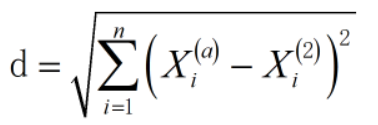## 4.案例实现

我们使用knn算法及其变种，对Pina印第安人的糖尿病进行预测。数据集可从下面下载。
链接：蓝奏云

### 4.1 导入相关库

# 导入相关模块
import numpy as np
from collections import Counter
import matplotlib.pyplot as plt
from sklearn.utils import shuffle
import pandas as pd

### 4.2 读取数据

#读取数据
print(data)

返回：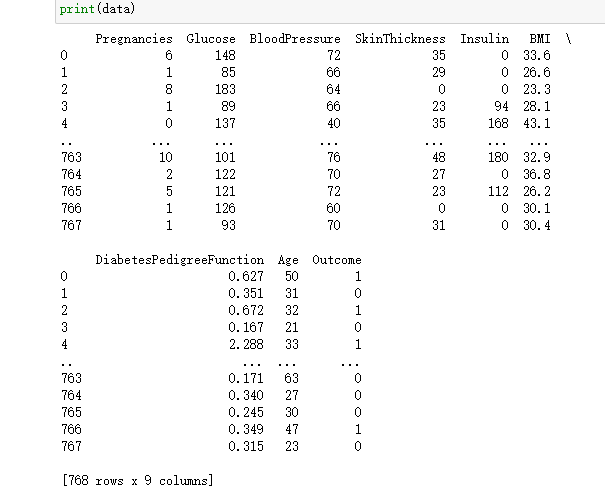### 4.3 读取变量名

label_need=data.keys()
print(label_need)

返回：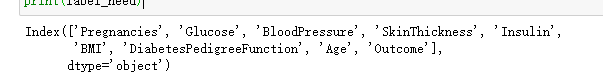### 4.4 定义X,Y数据

X = data[label_need].values[:,0:8]
y = data[label_need].values[:,8]
print(X)
print(y)

返回：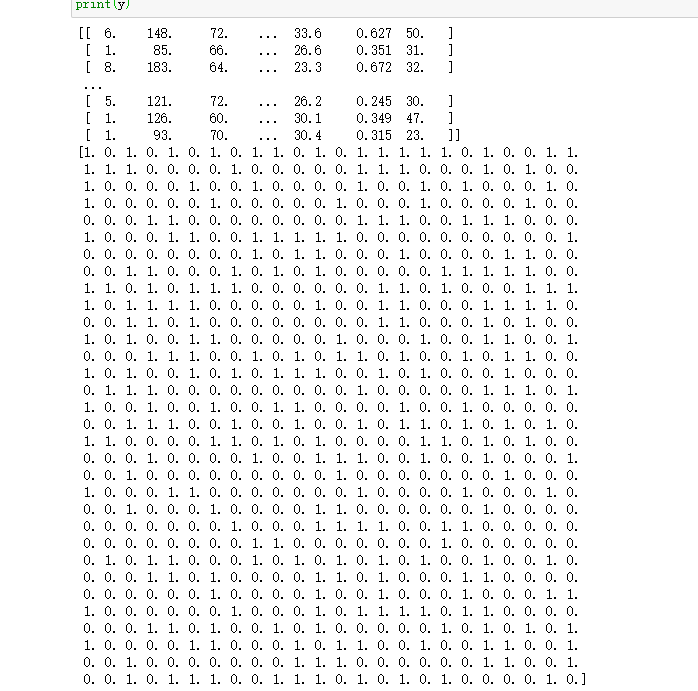### 4.5 分离训练集和测试集

from sklearn.model_selection import train_test_split
X_train, X_test, y_train,y_test = train_test_split(X, y, test_size=0.2)

# 打印训练集和测试集大小
print('X_train=', X_train.shape)
print('X_test=', X_test.shape)
print('y_train=', y_train.shape)
print('y_test=', y_test.shape)

返回：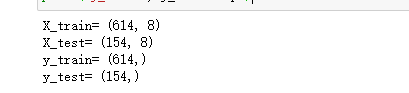### 4.6 计算欧式距离

# 测试实例样本量
num_test = X.shape
# 训练实例样本量
num_train = X_train.shape
# 基于训练和测试维度的欧氏距离初始化
dists = np.zeros((num_test, num_train))
# 测试样本与训练样本的矩阵点乘
M = np.dot(X, X_train.T)
# 测试样本矩阵平方
te = np.square(X).sum(axis=1)
# 训练样本矩阵平方
tr = np.square(X_train).sum(axis=1)
# 计算欧式距离
dists = np.sqrt(-2 * M + tr + np.matrix(te).T)
print(dists)

返回：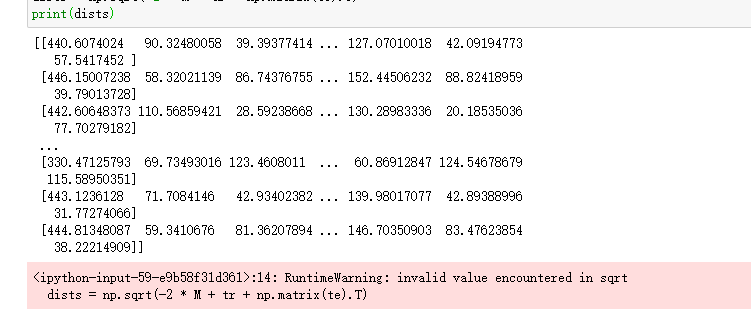### 4.7 可视化距离矩阵

dists = compute_distances(X_test, X_train)
plt.imshow(dists, interpolation='none')
plt.show()

返回：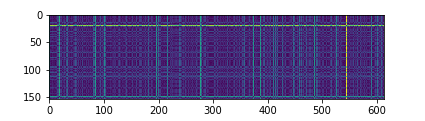### 4.8 预测样本

# 测试样本量
num_test = dists.shape
# 初始化测试集预测结果
y_pred = np.zeros(num_test)
# 遍历
for i in range(num_test):
# 初始化最近邻列表
closest_y = []
# 按欧氏距离矩阵排序后取索引，并用训练集标签按排序后的索引取值
# 最后拉平列表
# 注意np.argsort函数的用法
labels = y_train[np.argsort(dists[i, :])].flatten()
# 取最近的k个值
closest_y = labels[0:k]
# 对最近的k个值进行计数统计
# 这里注意collections模块中的计数器Counter的用法
c = Counter(closest_y)
# 取计数最多的那一个类别
y_pred[i] = c.most_common(1)
print(y_pred)

返回：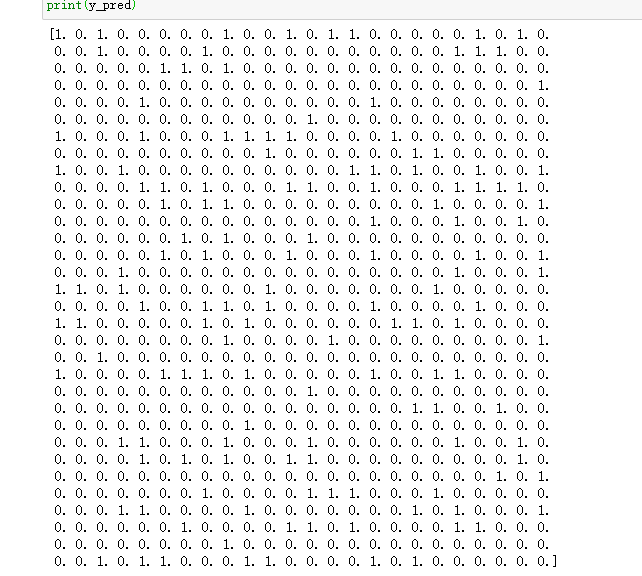### 4.9 查看正确率

查看实际和预测相符的个数：

# 找出预测正确的实例
num_correct = np.sum(y_test_pred == y_test)
print(num_correct)

返回：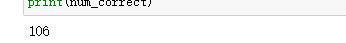计算正确率：

# 计算准确率
accuracy = float(num_correct) / X_test.shape
print('Got %d/%d correct=>accuracy:%f'% (num_correct, X_test.shape, accuracy))

返回：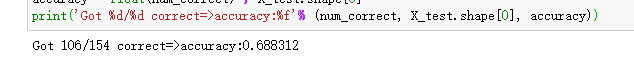### 4.10 交叉验证

# 折交叉验证
num_folds = 5
# 候选k值
k_choices = [1, 3, 5, 8, 10, 12, 15, 20, 50, 100]
X_train_folds = []
y_train_folds = []
# 训练数据划分
X_train_folds = np.array_split(X_train, num_folds)
# 训练标签划分
y_train_folds = np.array_split(y_train, num_folds)
k_to_accuracies = {}
# 遍历所有候选k值
for k in k_choices:
# 五折遍历
for fold in range(num_folds):
# 对传入的训练集单独划出一个验证集作为测试集
validation_X_test = X_train_folds[fold]
validation_y_test = y_train_folds[fold]
temp_X_train = np.concatenate(X_train_folds[:fold] + X_train_folds[fold + 1:])
temp_y_train = np.concatenate(y_train_folds[:fold] + y_train_folds[fold + 1:])
# 计算距离
temp_dists = compute_distances(validation_X_test, temp_X_train)
temp_y_test_pred = predict_labels(temp_y_train, temp_dists, k=k)
temp_y_test_pred = temp_y_test_pred.reshape((-1, 1))
# 查看分类准确率
num_correct = np.sum(temp_y_test_pred == validation_y_test)
num_test = validation_X_test.shape
accuracy = float(num_correct) / num_test
k_to_accuracies[k] = k_to_accuracies.get(k,[]) + [accuracy]


打印不同 k 值不同折数下的分类准确率：

# 打印不同 k 值不同折数下的分类准确率
for k in sorted(k_to_accuracies):
for accuracy in k_to_accuracies[k]:
print('k = %d, accuracy = %f' % (k, accuracy))

返回：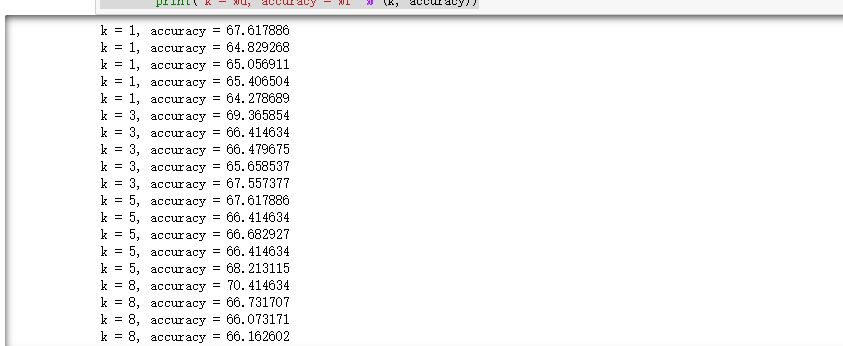不同 k 值不同折数下的分类准确率的可视化：

for k in k_choices:
# 取出第k个k值的分类准确率
accuracies = k_to_accuracies[k]
# 绘制不同k值准确率的散点图
plt.scatter([k] * len(accuracies), accuracies)
# 计算准确率均值并排序
accuracies_mean = np.array([np.mean(v) for k,v in sorted(k_to_accuracies.items())])
# 计算准确率标准差并排序
accuracies_std = np.array([np.std(v) for k,v in sorted(k_to_accuracies.items())])
# 绘制有置信区间的误差棒图
plt.errorbar(k_choices, accuracies_mean, yerr=accuracies_std)
# 绘图标题
plt.title('Cross-validation on k')
# x轴标签
plt.xlabel('k')
# y轴标签
plt.ylabel('Cross-validation accuracy')
plt.show()

返回：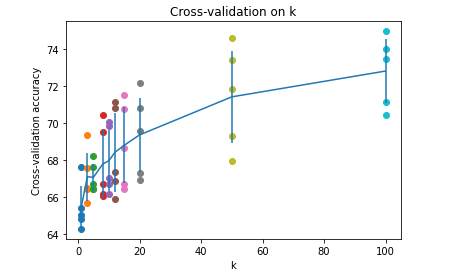### 5.1 对上述的再次实现：

# 导入KneighborsClassifier模块
from sklearn.neighbors import KNeighborsClassifier
# 创建k近邻实例
neigh = KNeighborsClassifier(n_neighbors=10)
# k近邻模型拟合
neigh.fit(X_train, y_train)
# k近邻模型预测
y_pred = neigh.predict(X_test)
# # 预测结果数组重塑
# y_pred = y_pred.reshape((-1, 1))
# 统计预测正确的个数
num_correct = np.sum(y_pred == y_test)
print(num_correct)
# 计算准确率
accuracy = float(num_correct) / X_test.shape
print('Got %d / %d correct => accuracy: %f' % (num_correct, X_test.shape, accuracy))

返回：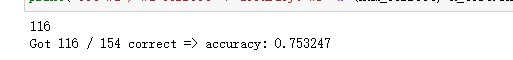### 5.2 另一种实现方式

5.2.1 加载数据

import pandas as pd
print('dataset shape {}'.format(data.shape))
data.info()

返回：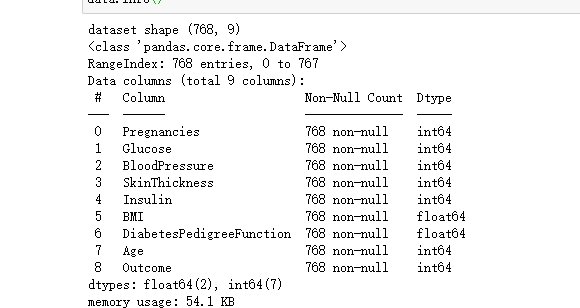5.2.2 分离训练集和测试集

X = data.iloc[:, 0:8]
Y = data.iloc[:, 8]
print('shape of X {}, shape of Y {}'.format(X.shape, Y.shape))

from sklearn.model_selection import train_test_split
X_train, X_test, Y_train,Y_test = train_test_split(X, Y, test_size=0.2)

返回：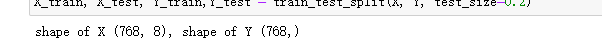5.2.3 模型比较

使用普通的knn算法、带权重的knn以及指定半径的knn算法分别对数据集进行拟合并计算评分

from sklearn.neighbors import KNeighborsClassifier, RadiusNeighborsClassifier

# 构建3个模型
models = []
models.append(('KNN', KNeighborsClassifier(n_neighbors=2)))
models.append(('KNN with weights', KNeighborsClassifier(n_neighbors=2, weights='distance')))

# 分别训练3个模型，并计算得分
results = []
for name, model in models:
model.fit(X_train, Y_train)
results.append((name, model.score(X_test, Y_test)))
for i in range(len(results)):
print('name: {}; score: {}'.format(results[i], results[i]))

返回：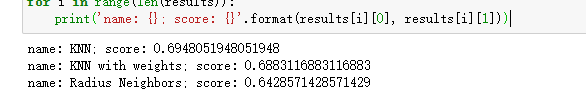问题来了，这个判断准确吗？ 答案是：不准确。

因为我们的训练集和测试集是随机分配的，不同的训练样本和测试样本组合可能导致计算出来的算法准确性有差异。

那么该如何解决呢？

我们可以多次随机分配训练集和交叉验证集，然后求模型评分的平均值。

scikit-learn提供了KFold和cross_val_score()函数来处理这种问题。

from sklearn.model_selection import KFold
from sklearn.model_selection import cross_val_score

results = []
for name, model in models:
kfold = KFold(n_splits=10)
cv_result = cross_val_score(model, X, Y, cv=kfold)
results.append((name, cv_result))

for i in range(len(results)):
print('name: {}; cross_val_score: {}'.format(results[i], results[i].mean()))

返回：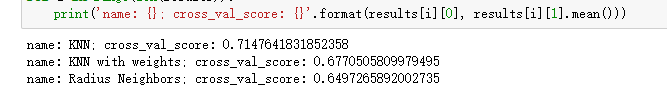上述代码，我们通过KFold把数据集分成10份，其中1份会作为交叉验证集来计算模型准确性，剩余9份作为训练集。cross_val_score()函数总共计算出10次不同训练集和交叉验证集组合得到的模型评分，最后求平均值。 看起来，还是普通的knn算法性能更优一些。

5.2.4 模型训练及分析

据上面模型比较得到的结论，我们接下来使用普通的knn算法模型对数据集进行训练，并查看对训练样本的拟合情况以及对测试样本的预测准确性情况：

knn = KNeighborsClassifier(n_neighbors=2)
knn.fit(X_train, Y_train)
train_score = knn.score(X_train, Y_train)
test_score = knn.score(X_test, Y_test)
print('train score: {}; test score : {}'.format(train_score, test_score))

返回：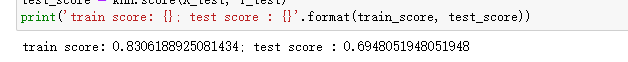从这里可以看到两个问题。

• 对训练样本的拟合情况不佳，评分才0.84多一些，说明算法模型太简单了，无法很好地拟合训练样本。
• 模型准确性不好，0.66左右的预测准确性。

我们画出曲线，查看一下。

我们首先定义一下这个画图函数，代码如下：

from sklearn.model_selection import learning_curve
import numpy as np

def plot_learning_curve(plt, estimator, title, X, y, ylim=None, cv=None,
n_jobs=1, train_sizes=np.linspace(.1, 1.0, 5)):
"""
Generate a simple plot of the test and training learning curve.

Parameters
----------
estimator : object type that implements the "fit" and "predict" methods
An object of that type which is cloned for each validation.

title : string
Title for the chart.

X : array-like, shape (n_samples, n_features)
Training vector, where n_samples is the number of samples and
n_features is the number of features.

y : array-like, shape (n_samples) or (n_samples, n_features), optional
Target relative to X for classification or regression;
None for unsupervised learning.

ylim : tuple, shape (ymin, ymax), optional
Defines minimum and maximum yvalues plotted.

cv : int, cross-validation generator or an iterable, optional
Determines the cross-validation splitting strategy.
Possible inputs for cv are:
- None, to use the default 3-fold cross-validation,
- integer, to specify the number of folds.
- An object to be used as a cross-validation generator.
- An iterable yielding train/test splits.

For integer/None inputs, if y is binary or multiclass,
:class:StratifiedKFold used. If the estimator is not a classifier
or if y is neither binary nor multiclass, :class:KFold is used.

Refer :ref:User Guide <cross_validation> for the various
cross-validators that can be used here.

n_jobs : integer, optional
Number of jobs to run in parallel (default 1).
"""
plt.title(title)
if ylim is not None:
plt.ylim(*ylim)
plt.xlabel("Training examples")
plt.ylabel("Score")
train_sizes, train_scores, test_scores = learning_curve(
estimator, X, y, cv=cv, n_jobs=n_jobs, train_sizes=train_sizes)
train_scores_mean = np.mean(train_scores, axis=1)
train_scores_std = np.std(train_scores, axis=1)
test_scores_mean = np.mean(test_scores, axis=1)
test_scores_std = np.std(test_scores, axis=1)
plt.grid()

plt.fill_between(train_sizes, train_scores_mean - train_scores_std,
train_scores_mean + train_scores_std, alpha=0.1,
color="r")
plt.fill_between(train_sizes, test_scores_mean - test_scores_std,
test_scores_mean + test_scores_std, alpha=0.1, color="g")
plt.plot(train_sizes, train_scores_mean, 'o--', color="r",
label="Training score")
plt.plot(train_sizes, test_scores_mean, 'o-', color="g",
label="Cross-validation score")

plt.legend(loc="best")
return plt

然后我们调用这个函数画一下图看看：

from sklearn.model_selection import ShuffleSplit

knn = KNeighborsClassifier(n_neighbors=2)
cv = ShuffleSplit(n_splits=10, test_size=0.2, random_state=0)
plt.figure(figsize=(10,6), dpi=200)
plot_learning_curve(plt, knn, 'Learn Curve for KNN Diabetes', X, Y, ylim=(0.0, 1.01), cv=cv)

返回：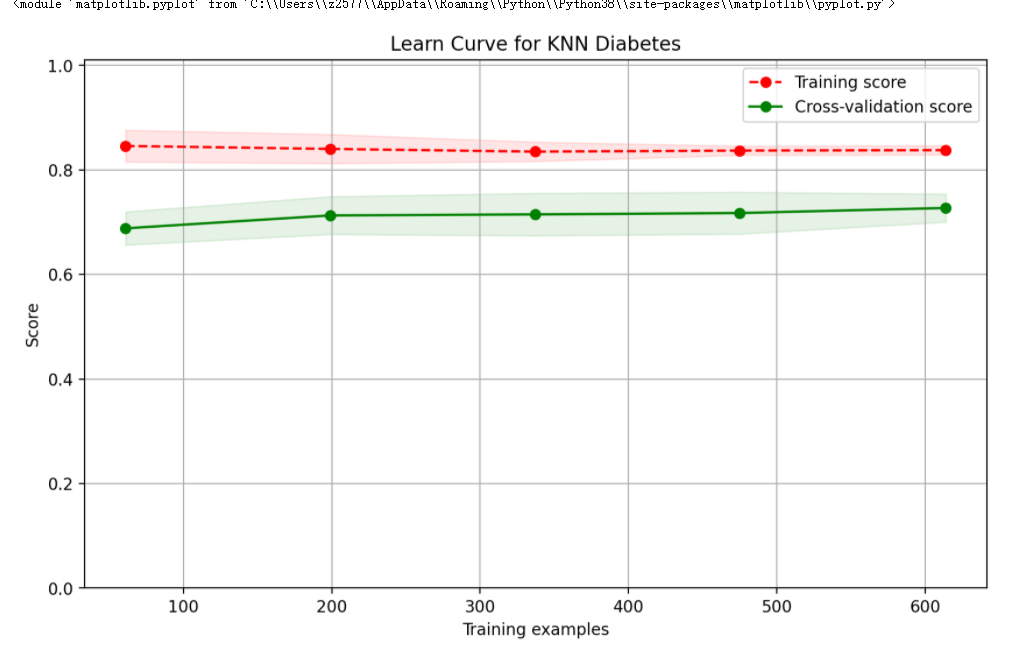展开全文近邻算法 python
• k近邻算法是机器学习中一种基础的分类与回归算法，通过输入新实例的特征向量，计算与新实例距离最为接近的k个训练集样本，之后在k个训练集样本中通过一定的选举策略确定出新实例的类别或者数值，从而达到分类或者...python 机器学习 人工智能
• 这个算法主要工作是测量不同特征值之间的距离，有个这个距离，就可以进行分类了。 简称kNN。 已知：训练集，以及每个训练集的标签。 接下来：和训练集中的数据对比，计算最相似的k个距离。选择相似数据中最多的那个...
• 自编Python代码实现了K近邻分类算法，并与sklearn结果做对比。python 分类 机器学习
•python 机器学习
• sample)) return distanceSet class KNN(): # k近邻算法 k = 0 def train(self, x_train, y_train): # train函数得到最好的k值，即以周围多少个点进行投票效果最好 Kmax = len(x_train) # k最大为点的个数 best_k =...机器学习 决策边界
• k近邻算法的介绍 k近邻算法是一种基本的分类和回归方法，这里只实现分类的k近邻算法k近邻算法的输入为实例的特征向量，对应特征空间的点；输出为实例的类别，可以取多类。 k近邻算法不具有显式的学习过程，...
• 一、算法简介k近邻法(k-nearest neighbor,k-NN)是一种基本的分类方法，输入的是实例的特征向量，对应于特征空间的点，输出结果为实例的类别，可以取多类。对于训练集来说，每个实例的类别已定，当分类时，对于新的...
• K近邻算法是依据新样本与k个与其相邻最近的样本的类别来进行分类的，所以K近邻算法不像前面所学习到的机器学习其他方法一样，将有标签的样本用来训练模型，而是直接计算新样本与所有样本点的距离来确定分类情况的。K...机器学习 python 分类
• kd树和寻找k近邻算法具体流程就不赘述了，这个链接写的很好懂https://zhuanlan.zhihu.com/p/23966698 按照链接里的算法写了k近邻的python实现 from math import sqrt class KDnode: def __init__(self, data, ...
• python K近近邻邻算算法法的的kd树树实实现现 这篇文章主要介绍了python K近邻算法的kd树实现小编觉得挺不错的现在分享给大家也给大家做个参考 一起跟随小编过 看看吧 k近近邻算算法法的的介介绍 k近邻算法是一种...
• group = array([[1.0,1.1],[1.0,1.0],[0,0],[0,0.1]]) #数组 labels = ['A','A','B','B'] #列表 return group, labels #k-近邻算法 def classify0(inX, dataSet, labels, k): dataSetSize = dataSet.shape ...机器学习 人工智能 最近邻分类算法 python
• 本文不设计k-近邻算法的原理，只通过python代码实现算法，并且没有用到机器学习库，根据算法流程一步一步实现。 代码实现 数据处理 假设我们已经获取了数据及其标签的文本文件，如下图所示。 数据有3个属性，标签...python 机器学习
• 什么是K-近邻算法？简单的说，K-近邻算法就是采用测量不同特征值之间的距离方法来进行分类。它的工作原理是：存在一个样本数据集合，也称作训练样本集，并且样本集中每个数据都存在标签，即我们知道样本集中每一数据...k近邻算法
• 机器学习中SVM、决策树、逻辑回归、K近邻算法python代码实现 上篇文章咱已经叙述了如何从excel表中读取保存的数据特征及类别标签，这篇文章主要介绍机器学习中常用到的算法python代码实现方式，主要包括SVM、决策树...python 决策树 机器学习 svm
• 机器学习_最近邻_k-近邻算法_python实现
• Python3实现的k近邻算法。数据集是约会网站的数据和手写数字的数据。根据《机器学习实战》中的例子改动而来。
• k-近邻算法 python可用版 直接可以用，我的博客里也有相关教程，欢迎关注
• Python环境下，使用MNIST数据集，实现KNN算法，对MNIST数据集中数据进行HOG特征提取，再进行预测，准确率较高...